Definitions
Grade expresses the incline of a length of track as a percentage (%). Grade for a straight length of track is independent of scale; a grade in N-scale is measured and calculated the same as a grade for HO, S, G, O, or even a full-size railroad.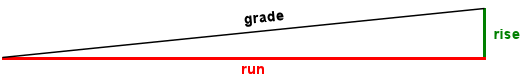Rise is the change in height from the beginning to end of the grade.

Run is the horizontal distance from the beginning to end of the grade.

The steeper the grade, the fewer cars a given locomotive can pull up the incline without stalling or slipping its wheels. As a general rule, grades on model railroad mainlines are typically limited to 2% or less. Branch lines are often steeper: 3%-5%. Certain special lines such as mining branches may be steeper still.

If grades are excessive, locomotives may have trouble pulling cars up the incline. This is true for both model railroads and full-size railroads. In both cases, steep grades can be managed by reducing the length of the train or using more than one locomotive per train. Sometimes that means two or more engines at the head of a train, but you can also use helper engines at the end or even in the middle of a train, just as real railroads do.

For a straight length of track, the formula for calculating the grade is: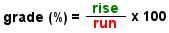Rise and run must use the same units of measure. For straight grades, you can use inches, millimeters, feet, miles, etc., as long as both rise and run use the same units. If you use inches, convert fractions to decimals. Example: 2 3/8 inches = 2.375 inches.

The formula divides rise by run, yielding a small number. The formula then multiplies by 100 to convert the number to a percent.

A grade rises 2.375 inches in a run of 165 inches. Dividing 2.375 by 165 = 0.01439. Multiply by 100 = 1.439% or slightly more than 1.4%.

Example 2 (a full size railroad):
A grade rises 578 feet in a run of 10 miles. First convert 10 miles to feet so that rise and run are in the same units:
10 x 5280 = 52800 feet.

Now use the formula.
578 divided by 52800 = 0.01094. Multiply by 100 = 1.094%. See? The formula for straight track works for any scale!

Railroad Boy Tip: It can sometimes be awkward to measure the run. Scenery and structures may interfere. Fortunately, your grade calculation will be accurate enough if you simply measure the track length from the beginning to the end of the grade. For the percentage grades we use in railroading, measuring the track length instead of the run introduces only a very small error — one you can safely ignore. It's easily good enough for (model) railroad work!

When a grade is on a curve, the curve exaggerates the effect of the grade, making it behave like a steeper incline.

As you might expect, the formula for calculating this effective grade on a curve is more complex, compared to the simple formula (above) for a straight grade. The calculation for effective grade depends both on the scale of your layout and on the radius of the curve.

When using these formulas for curved grades, the units for rise, run, and curve radius must be in inches.

For HO scale, the formula for a curved grade is: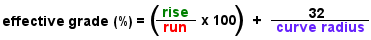For N-scale, the formula for a curved grade is: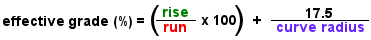These two formulas were empirically derived by model railroaders, including John Allen and John Armstrong, who actually measured the effort required to pull trains up curved grades as compared to pulling trains up straight grades.

NOTE: Both of these formulas for curved grades require that the units used in the calculations be in inches. The simple formula for a straight grade can use any units: millimeters, inches, feet, kilometers, miles, etc., but the formulas for grades on curves work only with inches.

Instructions

• Use either the HO or N-scale Grade Calculator, whichever is appropriate for your layout.
• Enter the rise and run in inches.
• If the grade is on a curve, enter the curve radius in inches. Otherwise leave curve radius blank.
• Enter only numbers; no symbols or units such as quotes or commas.
Fractions of an inch must be entered in decimal format:
1/8 = 0.125
1/4 = 0.25
3/8 = 0.375
1/2 = 0.5
5/8 = 0.625
3/4 = 0.75
7/8 = 0.875

Example: 2 7/8 = 2.875

After entering the rise, run, and (optionally) curve radius, click Calculate Effective Grade to perform the calculation.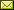Web site by DeTray Associates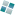www.DeTray.com